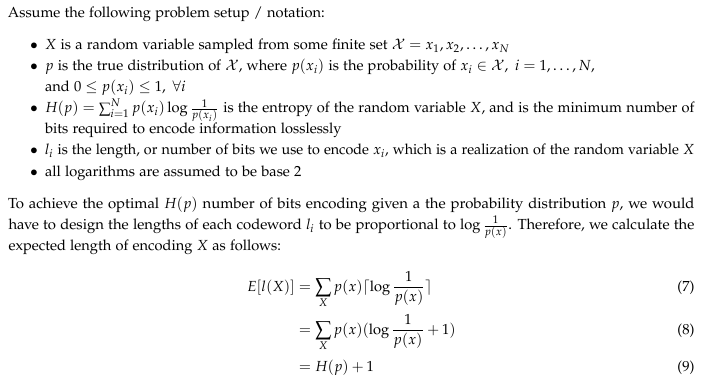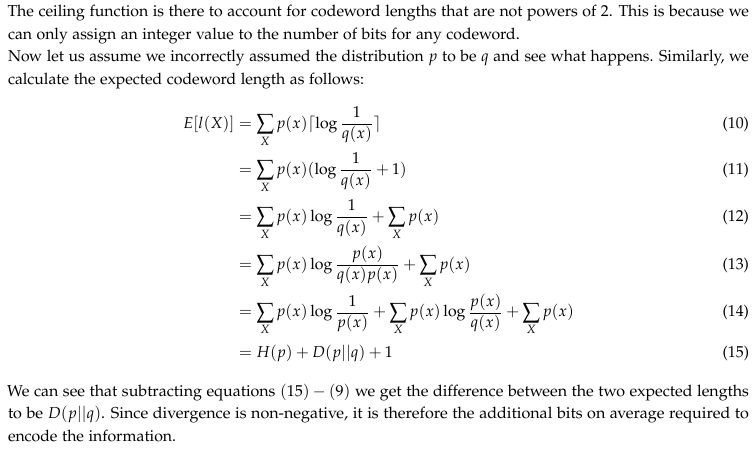# Kullback-Leibler Divergence Explained

Minimization of the KL-divergence can be thought of as maximizing the likelihood ratio, which appears in numerous applications.

Introduction
This blog is an introduction on the KL-divergence, aka relative entropy. The blog gives a simple example for understand relative entropy, and therefore I will not attempt to re-write the authors words. What I will do, in addition to reading the blog (which can be found at https://www.countbayesie.com/blog/2017/5/9/kullback-leibler-divergence-explained), is try to convey some extra information on top of this blog from my experience working in information theory.

Definition: Entropy
The blog introduces entropy with respect to a “probability distribution”. I want to argue that a better way to understand entropy, is that it is a measure of the “uncertainty” of a random variable, and not its probability distribution. The reason itself lies in the author’s next line: we can interpret entropy as “the minimum number of bits it would take us to encode our information”. A probability does not contain information, the information of which the author explains being encoded must come from some alphabet (in this case, a random variable), which has a probability distribution according to p(x_i). It is the random variable that contains the information, not the probability distribution.

In addition, quoting the same line from the author, entropy provides us with the “minimum number of bits it would take us to encoder our information”, I think it is important to mention that it is minimum number of bits it would take us to encode our information losslessly.

Definition: Relative Entropy
Relative entropy is a measure of the “distance” between two distributions. In statistics, it arises as an expected logarithm of the likelihood ratio. The relative entropy D(p||q) is a measure of the inefficiency of assuming that the distribution is q, when the true distribution is p.

It is not a true “distance” measure between the two distributions since it is not symmetric, i.e. D(p||q) =/= D(q||p), and does not satisfy the triangle inequality. The blog fails to mention the triangle inequality, although it may not be directly related to understanding the blog. The triangle inequality in this case would have D(p||q) <= D(p||u) + D(u||q), which does not hold in general.

An important property about relative entropy is its non-negativity, i.e. D(p||q) >= 0. Proof is attached below.Where equation (2) to (3) follows from Jensen’s inequality.

Why do we Optimize KL Divergence
In addition to the optimization of matching a binomial distribution example given in the blog, I will give another case where optimization of divergence may occur. Just a brief description on the contents of the blog, the author is essentially trying to solve the following problem:

p_optimal = argmin_{p} D(q||p)

Where q is the distribution obtained from the observations, and p is the binomial distribution. From the graph below, we can see that the minimum value of D(q||p) will give you the p that you should approximate your binomial distribution with.Note:

• The author is trying to approximate a binomial distribution to the observed data, hence D(q||p) is used
• This is not the conventional way of using divergence, because the author is basically treating the observations as the “true” distribution, which obviously is not true. The author’s intentions here is to approximate the observations with a simpler, more understood distribution, and therefore the use of KL divergence in this way may be a reasonable one
• Divergence is convex in the pair (q,p), hence the nice graph when performing optimization

From an information theory point of view, the divergence is the additional bits required to encode information losslessly. Let me demonstrate with the following example:Moving on to Machine Learning Related Applications
The author mentions two applications of optimizing divergence: variational autoencoders and variational Bayesian methods. The author does not explain these applications, but do provide links to learn more about them:

I will just briefly describe the intuition of minimizing divergence in machine learning applications. Generative models in machine learning often involves generating models of distributions that reflect reality as closely as possible. For example, applications of Generative Adversarial Networks on pictures often perform tasks such as generating a colored picture from a black-and-white image to look as real as possible. In applications such as these, the inputs are often images, or pixels. The network will learn the dependencies between the pixels, and use this to create images that look real, e.g., neighboring pixels usually have similar color. Therefore, the generator is trying to minimize the divergence between its learnt pixel distribution and the pixel distribution of a real image.

Finally, I want to say that minimization of the KL-divergence can be thought of as maximizing the likelihood ratio, which appears in numerous applications. A simple way to see it is through the following:Hopefully, I was able to provide you with some supplementary material on the tutorial of KL_divergence, aka relative entropy provided by the author of the blog. I think the importance of KL-divergence lies in its ability to quantify how far off your estimation of a distribution may be from the true distribution. In the case of the blog, if we assumed the observed data to be drawn from a distribution parameterized by some parameter set, what should the parameters be to most closely represent the observed data.

Finally, if you are interested in learning about variations of KL-divergence, I recommend looking into the Jesen-Shannon divergence, which is a symmetric divergence and measures the similarity between two distributions. Another is the Renyi divergence, which is a generalization of the K-L divergence and mostly found in applications of quantum physics.

Author: Joshua Chou | Editor: Qintong Wu